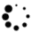``````x = [4,1,2,3]
y = sorted(x)       # 结果是[1,2,3,4]，但x没有变
x.sort()            # x变为[1,2,3,4]

``````

``````# 通过绝对值对列表元素从最大到最小排序
x = sorted([-4,1,-2,3], key=abs, reverse=True) # 是[-4,3,-2,1]

# 从最高数到最低数排序单词和计数
wc = sorted(word_counts.items(),
key=lambda (word, count): count,
reverse=True)

``````

``````even_numbers = [x for x in range(5) if x % 2 == 0]     # [0, 2, 4]
squares      = [x * x for x in range(5)]               # [0, 1, 4, 9, 16]
even_squares = [x * x for x in even_numbers]           # [0, 4, 16]

``````

``````square_dict = { x : x * x for x in range(5) }    # { 0:0, 1:1, 2:4, 3:9, 4:16}
square_set  = { x * x for x in [1, -1] }         # { 1 }

``````

``````zeroes = [0 for _ in even_numbers]    # 和even_numbers有相同的长度

``````

``````pairs = [(x, y)
for x in range(10)
for y in range(10)]    # 100个对(0,0) (0,1) ... (9,8), (9,9)

``````

``````increasing_pairs = [(x, y)                       # 只考虑x < y的对
for x in range(10)           # range(lo, hi) 与之相等
for y in range(x + 1, 10)]   # [lo, lo + 1, ..., hi - 1]

``````

``````def lazy_range(n):
"""a lazy version of range"""
i = 0
while i < n:
yield i
i += 1

``````

``````for i in lazy_range(10):
do_something_with(i)

``````

（Python 确实有一个和 `lazy_range` 一样的函数，叫作 `xrange`，并且在 Python 3 中，`range` 函数本身就是延迟的。）这意味着，你甚至可以创建一个无限的序列：

``````def natural_numbers():
"""returns 1, 2, 3, ..."""
n = 1
while True:
yield n
n += 1

``````延迟的缺点是，你只能通过生成器迭代一次。如果需要多次迭代某个对象，你就需要每次都重新创建一个生成器，或者使用列表。

``````lazy_evens_below_20 = (i for i in lazy_range(20) if i % 2 == 0)

``````

``````import random

four_uniform_randoms = [random.random() for _ in range(4)]

# [0.8444218515250481,
#  0.7579544029403025,
#  0.420571580830845,
#  0.25891675029296335]
# random.random()生成在0-1之间均匀分布的随机数，是最常用的随机函数

``````

`random` 模块实际上生成的是基于一种内部状态的确定性的伪随机数。如果你想得到可复生的结果，可以用 `random.seed` 生成随机数种子：

``````random.seed(10)          # 设置随机数种子为10
print random.random()    # 0.57140259469
random.seed(10)          # 重设随机数种子为10
print random.random()    # 再次得到0.57140259469

``````

``````random.randrange(10)    # 从range(10) = [0, 1, ..., 9]中随机选取
random.randrange(3, 6)  # 从range(3, 6) = [3, 4, 5]中随机选取

``````

``````up_to_ten = range(10)
random.shuffle(up_to_ten)
print up_to_ten
# [2, 5, 1, 9, 7, 3, 8, 6, 4, 0]，（你的结果可能不同）

``````

``````my_best_friend = random.choice(["Alice", "Bob", "Charlie"])    # 对我来说是"Bob"

``````

``````lottery_numbers = range(60)
winning_numbers = random.sample(lottery_numbers, 6)  # [16, 36, 10, 6, 25, 9]

``````

``````four_with_replacement = [random.choice(range(10))
for _ in range(4)]
# [9, 4, 4, 2]

``````

``````import re

print all([                                 # 所有这些语句都为true，因为
not re.match("a", "cat"),               # * 'cat'不以'a'开头
re.search("a", "cat"),                  # * 'cat'里有一个字符'a'
not re.search("c", "dog"),              # * 'dog'里没有字符'c'
3 == len(re.split("[ab]", "carbs")),    # * 分割掉a,b，剩余长度为3
"R-D-" == re.sub("[0-9]", "-", "R2D2")  # 用虚线进行位的替换
] # 打印True

``````

``````# 按惯例，我们给下面的类起个PascalCase的名字
class Set:

# 这些是成员函数
# 每个函数都取第一个参数"self"（另一种惯例）
# 它表示所用到的特别的集合对象

def __init__(self, values=None):
"""This is the constructor.
It gets called when you create a new Set.
You would use it like
s1 = Set()           # 空集合
s2 = Set([1,2,2,3])  # 用值初始化"""

self.dict = {} # Set的每一个实例都有自己的dict属性
# 我们会用这个属性来追踪成员关系
if values is not None:
for value in values:

def __repr__(self):
"""this is the string representation of a Set object
if you type it at the Python prompt or pass it to str()"""
return "Set: " + str(self.dict.keys())

# 通过成为self.dict中对应值为True的键，来表示成员关系
self.dict[value] = True

# 如果它在字典中是一个键，那么在集合中就是一个值
def contains(self, value):
return value in self.dict

def remove(self, value):
del self.dict[value]

``````

``````s = Set([1,2,3])
print s.contains(4)       # True
s.remove(3)
print s.contains(3)       # False

``````

``````def exp(base, power):
return base ** power

``````

``````def two_to_the(power):
return exp(2, power)

``````

``````from functools import partial
two_to_the = partial(exp, 2)      # 现在是一个包含一个变量的函数
print two_to_the(3)               # 8

``````

``````square_of = partial(exp, power=2)
print square_of(3)                  # 9

``````

``````def double(x):
return 2 * x

xs = [1, 2, 3, 4]
twice_xs = [double(x) for x in xs]         # [2, 4, 6, 8]
twice_xs = map(double, xs)                 # 和上面一样
list_doubler = partial(map, double)        # double了一个列表的*function*
twice_xs = list_doubler(xs)                # 同样是[2, 4, 6, 8]

``````

``````def multiply(x, y): return x * y

products = map(multiply, [1, 2], [4, 5]) # [1 * 4, 2 * 5] = [4, 10]

``````

``````def is_even(x):
"""True if x is even, False if x is odd"""
return x % 2 == 0

x_evens = [x for x in xs if is_even(x)]       # [2, 4]
x_evens = filter(is_even, xs)                 # 和上面一样
list_evener = partial(filter, is_even)        # filter了一个列表的*function*
x_evens = list_evener(xs)                     # 同样是[2, 4]

``````

`reduce` 结合了列表的头两个元素，它们的结果又结合了列表的第 3 个元素，这个结果之后又结合了第 4 个元素，依次下去，直到得到一个单独的结果：

``````x_product = reduce(multiply, xs)              # = 1 * 2 * 3 * 4 = 24
list_product = partial(reduce, multiply)      # reduce了一个列表的*function*
x_product = list_product(xs)                  # 同样是24

``````

``````# 非Python用法
for i in range(len(documents)):
document = documents[i]
do_something(i, document)

# 也非Python用法
i = 0
for document in documents:
do_something(i, document)
i += 1

``````

Python 惯用的解决方案是使用枚举（`enumerate`），它会产生 `(index, element)` 元组：

``````for i, document in enumerate(documents):
do_something(i, document)

``````

``````for i in range(len(documents)): do_something(i)     # 非Python用法
for i, _ in enumerate(documents): do_something(i)   # Python用法

``````

``````list1 = ['a', 'b', 'c']
list2 = [1, 2, 3]
zip(list1, list2)         # 是[('a', 1), ('b', 2), ('c', 3)]

``````

``````pairs = [('a', 1), ('b', 2), ('c', 3)]
letters, numbers = zip(*pairs)

``````

``````zip(('a', 1), ('b', 2), ('c', 3))

``````

``````def add(a, b): return a + b

``````

``````def doubler(f):
def g(x):
return 2 * f(x)
return g

``````

``````def f1(x):
return x + 1

g = doubler(f1)
print g(3)           # 8 (== ( 3 + 1) * 2)
print g(-1)          # 0 (== (-1 + 1) * 2)

``````

``````def f2(x, y):
return x + y

g = doubler(f2)
print g(1, 2)    # TypeError: g()只能有一个参数（给定了两个）

``````

``````def magic(*args, **kwargs):
print "unnamed args:", args
print "keyword args:", kwargs

magic(1, 2, key="word", key2="word2")

# 输出
# 未命名args: (1, 2)
# 关键词args: {'key2': 'word2', 'key': 'word'}

``````

``````def other_way_magic(x, y, z):
return x + y + z

x_y_list = [1, 2]
z_dict = { "z" : 3 }
print other_way_magic(*x_y_list, **z_dict)    # 6

``````

``````def doubler_correct(f):
"""works no matter what kind of inputs f expects"""
def g(*args, **kwargs):
"""whatever arguments g is supplied, pass them through to f"""
return 2 * f(*args, **kwargs)
return g

g = doubler_correct(f2)
print g(1, 2) # 6

``````30:00